Get instant live expert help with Excel or Google Sheets“My Excelchat expert helped me in less than 20 minutes, saving me what would have been 5 hours of work!”

#### Post your problem and you'll get expert help in seconds

Your message must be at least 40 characters
Our professional experts are available now. Your privacy is guaranteed.

# How to Use the COS Function in Excel

Cosine is one of the basic functions in Trigonometry and manual calculations are generally hard to perform.  Excel provides an easy way of calculating the cosine through the COS function.  This tutorial will assist all levels of Excel users in the usage and syntax of COS function.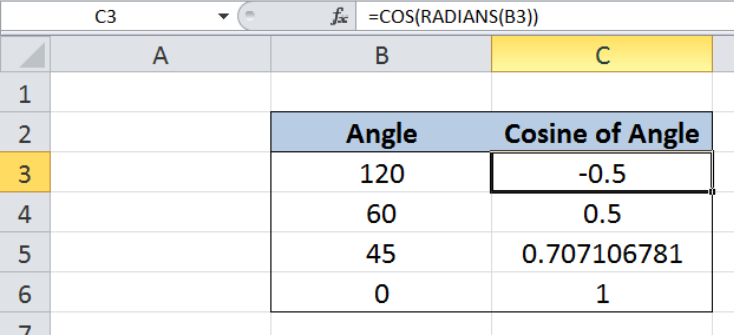Figure 1.  Final result: Excel COS function

Formula 1: `=COS(RADIANS(B3))`

Formula 2: `=COS(B3*PI()/180)`

## Syntax of COS Function

COS function returns the cosine of an angle

`=COS(number)`

• number – an angle in radians for which we want the cosine
• If an angle is given in degrees, convert it to radians by using the RADIANS function or multiplying the angle by PI()/180

`=RADIANS(angle)`

• angle – an angle in degrees that we want to convert to radians

## Setting up our Data

Our table contains two columns: Angle (column B) and Cosine of Angle (column C).  We want to determine the cosine of the angles in column B by using the COS function.  The results will be recorded in column D.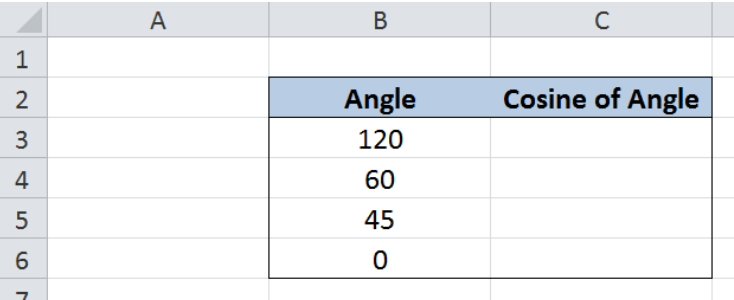Figure 2.  Sample data for Excel COS function

The COS function calculates the cosine of an angle in radians.  Since angles are normally given in degrees form, we have to convert the angle into radians first.  There are two ways to do this:

• by using the RADIANS function
• by multiplying the angle in degrees by PI()/180

## Calculate cosine of angle converted through RADIANS

We want to calculate the cosine of an angle by using the COS function in combination with the RADIANS function.  Let us follow these steps:

Step 1.  Select cell C3

Step 2.  Enter the formula: `=``COS(RADIANS(B3))`

Step 3.  Press ENTER

Step 4:  Copy the formula in cell C3 to cells C4:C6 by clicking the “+” icon at the bottom-right corner of cell C3 and dragging it down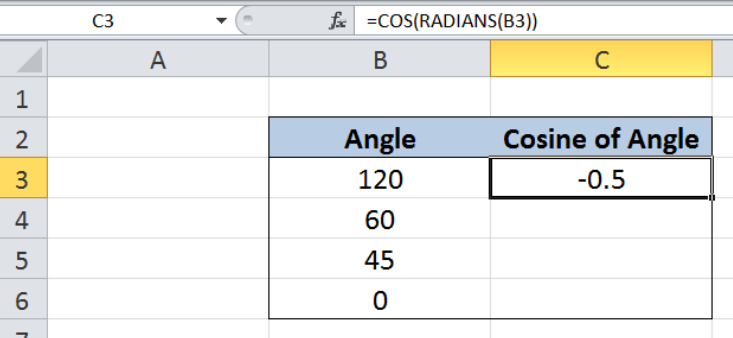Figure 3.  Entering the formula using COS and RADIANS

Our formula first converts the angle in degrees into radians through the RADIANS function, then calculates the cosine of the angle that is now in radians.  As a result, the value in cell C3 is -0.5.

Below table shows the cosine of the angles using the COS and RADIANS functions.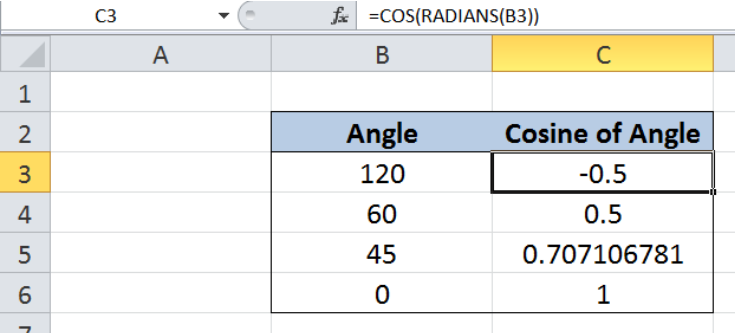Figure 4.  Cosine of angles calculated using COS and RADIANS

## Calculate cosine of angle converted by PI()/180

The alternative to converting angles in degrees into radians is by multiplying the angle with PI()/180.  In order to calculate the cosine of an angle by using the COS function in combination with the conversion factor, let us follow these steps:

Step 1.  Select cell C3

Step 2.  Enter the formula: `=``COS(B3*PI()/180)`

Step 3.  Press ENTER

Step 4:  Copy the formula in cell C3 to cells C4:C6 by clicking the “+” icon at the bottom-right corner of cell C3 and dragging it down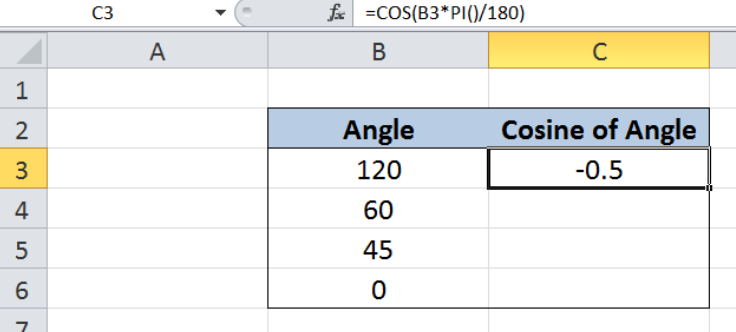Figure 5.  Entering the formula using COS and conversion factor

Our formula converts the angle in degrees into radians through the conversion factor, then calculates the cosine of the angle through the COS function.  As a result, the value in cell C3 is -0.5, which is the same as in the previous example.

The table below shows the cosine of the angles using the COS and the conversion factor “PI()/180”.  As we can see, the resulting cosine values are the same as in the first method using COS and RADIANS.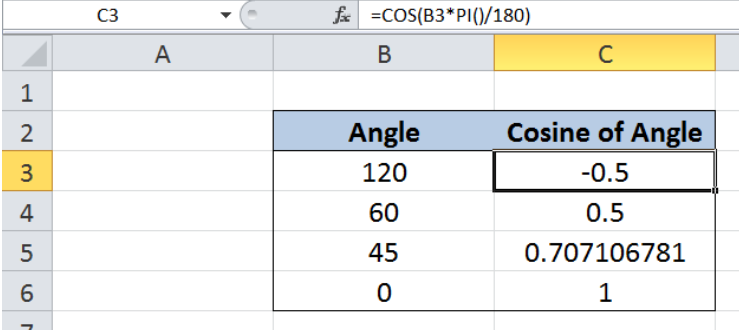Figure 6.  Cosine of angles calculated using COS and conversion factor

Most of the time, the problem you will need to solve will be more complex than a simple application of a formula or function. If you want to save hours of research and frustration, try our live Excelchat service! Our Excel Experts are available 24/7 to answer any Excel question you may have. We guarantee a connection within 30 seconds and a customized solution within 20 minutes.

### Did this post not answer your question? Get a solution from connecting with the expert.Another blog reader asked this question today on Excelchat:## Subscribe to Excelchat.coAnother blog reader asked this question today on Excelchat: